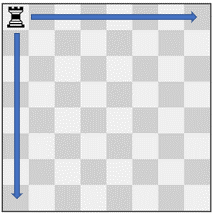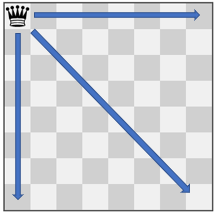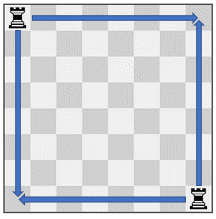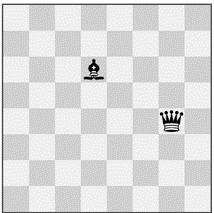##### Welcome to Subscribe On Youtube

Formatted question description: https://leetcode.ca/all/2056.html

# 2056. Number of Valid Move Combinations On Chessboard (Hard)

There is an 8 x 8 chessboard containing n pieces (rooks, queens, or bishops). You are given a string array pieces of length n, where pieces[i] describes the type (rook, queen, or bishop) of the ith piece. In addition, you are given a 2D integer array positions also of length n, where positions[i] = [ri, ci] indicates that the ith piece is currently at the 1-based coordinate (ri, ci) on the chessboard.

When making a move for a piece, you choose a destination square that the piece will travel toward and stop on.

• A rook can only travel horizontally or vertically from (r, c) to the direction of (r+1, c), (r-1, c), (r, c+1), or (r, c-1).
• A queen can only travel horizontally, vertically, or diagonally from (r, c) to the direction of (r+1, c), (r-1, c), (r, c+1), (r, c-1), (r+1, c+1), (r+1, c-1), (r-1, c+1), (r-1, c-1).
• A bishop can only travel diagonally from (r, c) to the direction of (r+1, c+1), (r+1, c-1), (r-1, c+1), (r-1, c-1).

You must make a move for every piece on the board simultaneously. A move combination consists of all the moves performed on all the given pieces. Every second, each piece will instantaneously travel one square towards their destination if they are not already at it. All pieces start traveling at the 0th second. A move combination is invalid if, at a given time, two or more pieces occupy the same square.

Return the number of valid move combinations.

Notes:

• No two pieces will start in the same square.
• You may choose the square a piece is already on as its destination.
• If two pieces are directly adjacent to each other, it is valid for them to move past each other and swap positions in one second.

Example 1:Input: pieces = ["rook"], positions = [[1,1]]
Output: 15
Explanation: The image above shows the possible squares the piece can move to.


Example 2:Input: pieces = ["queen"], positions = [[1,1]]
Output: 22
Explanation: The image above shows the possible squares the piece can move to.


Example 3:Input: pieces = ["bishop"], positions = [[4,3]]
Output: 12
Explanation: The image above shows the possible squares the piece can move to.


Example 4:Input: pieces = ["rook","rook"], positions = [[1,1],[8,8]]
Output: 223
Explanation: There are 15 moves for each rook which results in 15 * 15 = 225 move combinations.
However, there are two invalid move combinations:
- Move both rooks to (8, 1), where they collide.
- Move both rooks to (1, 8), where they collide.
Thus there are 225 - 2 = 223 valid move combinations.
Note that there are two valid move combinations that would result in one rook at (1, 8) and the other at (8, 1).
Even though the board state is the same, these two move combinations are considered different since the moves themselves are different.


Example 5:Input: pieces = ["queen","bishop"], positions = [[5,7],[3,4]]
Output: 281
Explanation: There are 12 * 24 = 288 move combinations.
However, there are several invalid move combinations:
- If the queen stops at (6, 7), it blocks the bishop from moving to (6, 7) or (7, 8).
- If the queen stops at (5, 6), it blocks the bishop from moving to (5, 6), (6, 7), or (7, 8).
- If the bishop stops at (5, 2), it blocks the queen from moving to (5, 2) or (5, 1).
Of the 288 move combinations, 281 are valid.


Constraints:

• n == pieces.length
• n == positions.length
• 1 <= n <= 4
• pieces only contains the strings "rook""queen", and "bishop".
• There will be at most one queen on the chessboard.
• 1 <= xi, yi <= 8
• Each positions[i] is distinct.

Companies:

Related Topics:
Array, String, Backtracking, Simulation

## Solution 1. Backtracking

Time Complexity:

For each DFS level, the piece can most move to 4 * 7 + 1 = 29 different positions. There are at most 4 levels. So there are O(29^4) different combination of positions.

For each combination, the valid function at most check 1 + 2 + 3 = 6 pairs of pieces, and each pair takes takes at most 7 steps. So the valid function takes O(6 * 7) times for each combination.

So, overall, this solution takes O(29^4 * 6 * 7) time.

This number looks large but since lots of combinations are skipped, it can get accepted.

// OJ: https://leetcode.com/problems/number-of-valid-move-combinations-on-chessboard/
// Time: O(29^4 * 6 * 7)
// Space: O(64)
class Solution {
public:
int countCombinations(vector<string>& pc, vector<vector<int>>& pos) {
int N = pc.size();
vector<array<int, 2>> dirs = { { {0,1},{0,-1},{1,0},{-1,0} }, { {1,1},{1,-1},{-1,1},{-1,-1} }, { {0,1},{0,-1},{1,0},{-1,0},{1,1},{1,-1},{-1,1},{-1,-1} } }, goal;
bool used = {};
auto getDelta = [](int from, int to) { return from < to ? 1 : (from > to ? -1 : 0); };
auto valid = [&]() {
int last = goal.size() - 1;
for (int i = last - 1; i >= 0; --i) {
int x1 = pos[last] - 1, y1 = pos[last] - 1, x1g = goal[last], y1g = goal[last], dx1 = getDelta(x1, x1g), dy1 = getDelta(y1, y1g);
int x2 = pos[i] - 1, y2 = pos[i] - 1, x2g = goal[i], y2g = goal[i], dx2 = getDelta(x2, x2g), dy2 = getDelta(y2, y2g);
while (x1 != x1g || y1 != y1g || x2 != x2g || y2 != y2g) {
if (x1 != x1g) x1 += dx1;
if (y1 != y1g) y1 += dy1;
if (x2 != x2g) x2 += dx2;
if (y2 != y2g) y2 += dy2;
if (x1 == x2 && y1 == y2) return false;
}
}
return true;
};
function<int(int)> dfs = [&](int i) {
if (i == N) return 1;
int x = pos[i] - 1, y = pos[i] - 1, dir = pc[i] == "rook" ? 0 : (pc[i] == "bishop" ? 1 : 2), ans = 0;
if (!used[x][y]) {
used[x][y] = true;
goal.push_back({x, y});
if (valid()) ans += dfs(i + 1);
goal.pop_back();
used[x][y] = false;
}
for (auto &[dx, dy] : dirs[dir]) {
int a = x, b = y;
do {
a += dx;
b += dy;
if (a < 0 || a > 7 || b < 0 || b > 7) break;
if (used[a][b]) continue;
used[a][b] = true;
goal.push_back({a, b});
if (valid()) ans += dfs(i + 1);
goal.pop_back();
used[a][b] = false;
} while (true);
}
return ans;
};
return dfs(0);
}
};